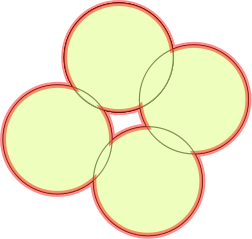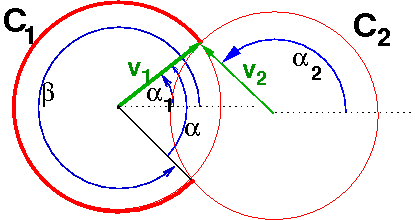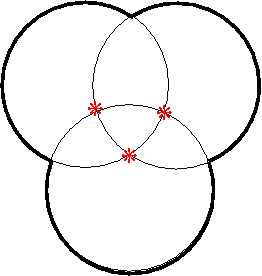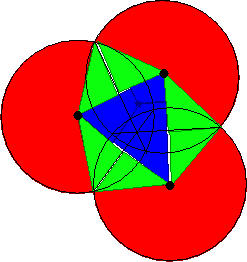# Mass Properties of the Union of Circles

## Abstract

We compute the exterior perimeter length and area of the union of N congruent circles. The expected time is linear in N. The computation is exact to within floating point error.

This page is still preliminary, and may have some errors. However, I believe that they are correctable

# Introduction

This work is a dry run before computing mass properties, such as surface area and volume, of the union of a number of spheres in E3. The application is computing the interference between two proteins, each expressed as the union of a number of spherical atoms.

# Notation and Definitions

1. π is pi. (It's not recognizable in some fonts.)
2. α and β are greek lowercase alpha and beta. (They're not recognizable as greek in some fonts.)
3. The visible perimeter of the union of some circles is the total arc length of the circles' perimeters that is not inside any other circle. That's the red line in the following Figure.The former name for visible perimeter was exterior perimeter but that was misleading.
4. norm(θ) is θ reduced to the range -π<θ≤π
5. σ(x) is the signum of x, that is, -1, 0, or 1, depending on the sign of x.
6. In this work, all circles and spheres have unit diameter. This shouldn't affect the theory, but helps the implementation considerably.

# My Prior Art

## Linear Object Space Hidden Surfaces

This is an algorithm and implementation from about 1980 for taking N overlapping circles, i.e., projected spheres, and finding the visible arcs of the ones in front. In 1980 could process 10,000 circles in under 400 seconds. The host was probably 0.5 MIPS and, IIRC, had 256KB of core memory.

## UNION2, UNION3, and Boolean Operations and Their Mass Properties

UNION2 is a demo program that finds the area and perimeter of the union of many random equal-sized squares. For example, for 100,000,000 squares with edge length 0.0006, the cpu execution time on a 1600 mhz pentium was only 11 minutes.

UNION3 is a fast algorithm for computing the volume, area, and other mass properties, of the union of many polyhedra. UNION3 is well suited for parallel machines. A prototype implementation for identical random cubes has processed 20,000,000 polyhedra, containing half a billion subfacets, on a 2.4GHz (?) dual processor Pentium Xeon workstation in about one hour.

See Union.

## Geometric Operations on Millions of Objects

The slides of this good summary talk, which describes the general geometric ideas used here, are here.

## Comparison of current work to earlier work

1. N is much smaller here - perhaps 1000 instead of thousands or

millions.

1. Memory will not be a problem here.
2. Packaged data

structures will be feasible.

1. Implementation will be easier.
2. While circles and spheres appear simpler than cubes, analyzing

their intersections has its interesting points.

# Mathematical Ideas

1. To compute the perimeter length of the union of two circles, it is sufficient to know where they intersect. Consider the following figure:Two Intersecting Circles
The perimeter of circle C1 that contributes to the total is that part that is outside the other circle, that is, β-α. This requires the angles of the intersections of C1 with the other circle.
2. Conceptually we would process the arcs as follows.
1. Initialize P, the total visible perimeter, to be 0.
2. Iterate thru the visible arcs.
3. For each visible arcs, find the angles of start and end, s and e.
4. Update P' = P + e - s
3. Since each visible arc's ends occur at visible intersections, and each visible intersection induces two ends of visible arcs, in fact, we interate thru the visible intersections. For each visible intersection, we determine two angles and add or subtract them from the running total for the visible perimeter.
4. One angle will be added and the other subtracted. Which is which?
5. Whether α1 is added or subtracted is determined from the z component of the cross product of the vectors v1 and v2 . Add the angle iff this component is negative.
6. IOW:
1. Let the two angles be α1 and α2 ; see the figure.
2. Compute p = σ(norm(α12)) (α12)
3. Add p to the subtotal for the visible perimeter.
7. C may have many intersections with other circles. Only those intersections not contained in other circles contribute to the visible perimeter. In this Figure:Hidden Intersections are Irrelevant
the three starred intersections should be ignored.
8. Therefore a first pass at an algorithm to compute the visible perimeter P goes as follows.
2. Find X, the set of intersections of any two circles in S. (Each pair of intersecting circles will produce two points.)
3. Test each member x of X against all the other N-2 circles of S.
4. If any such circle contains x, then delete it from X.
5. Note that each remaining x is an endpoint of two arcs, one on each of the two circles whose intersection produced x.
6. Compute an α and β for each x (one for each arc), and add or subtract to the subtotal for P.
9. There are two special cases to consider:
1. What if C is completely visible but has no intersections with other circles? In that case, must be added to the total perimeter.
2. What if the visible perimeter of C contains the positive x-axis? In that case, β-α will be negative, and must be increased by
We will handle these cases together as follows.
1. Consider the point z on C with angle 0 (relative to C's center).
2. Iff z is contained in any other circle, then add to the visible perimeter total.

# Efficient Implementation

The above algorithm has time N3 in the worst case, which occurs when many circles overlap each other. For circles representing molecules, the time would probably be N2 because we would expect a linear number of actual circle intersections We will reduce this expected time down to N. Our technique is to use a uniform grid, as described in the prior art above.

1. Overlay a uniform grid on the data. The grid cell size should be at least double the circle size. The exact value is not that important.
2. For each circle, find what cells it completely contains, and mark them as covered cells.
3. For each cell that is not covered, initialize an empty overlap set that will contain circles whose perimeters pass thru it.
4. For each circle c, find what cells its perimeter passes thru. Add c to those cells' overlap sets.
5. Initialize the subtotal for the visible perimeter P to zero.
6. For each cell g that is not covered, compare all the pairs of circles in g 's overlap set to find all intersections x.
1. For each x, test it against all the other circles in g 's overlap set to see if any contain x. If any do then do not consider x to be an intersection.
2. For each remaining x, find the angles of the two vectors from the centers of the two circles who caused x, compute two angles, one positive and the other negative, and add them to P.
7. For each circle c, compute its 0-angle point z.
1. Find the cell g containing z.
2. If g is not a covered cell, then test z against all the circles in g.
3. If z is not in any circle or covered cell, then add to P.

# Notes

1. We never compute the explicit visible arcs since we compute each arc's end angles at different times. That's the beauty of these local algorithms.
2. However, that all falls apart if we miss any ends. Therefore there must be no topological errors during the computations. Whether the intersection of two circles' perimeters is inside or outside another circle must always be determined correctly.
3. Degeneracies, such as three circles intersecting at a point, must be handled. I recommend Simulation of Simplicity.
4. The covered cell concept induces sparsity in the data structure. Because of it, the expected number of circles per cell remains constant as N grows to infinity.
5. The expected number of visible circle intersections is linear even tho the total number is quadratic.
6. The time to test whether each intersection is visible is constant.
7. Therefore the total time is linear in the number of circles.

# Computing the Union Area

This section is preliminary, and may have errors.

The area of the union of circles has three components as shown in this figure.Area of the Union of Three Circles
1. There is one red component for each visible arc. It is the sector defined by that arc and its circle's center.
We will compute it in two pieces, one depending on each end.
2. There are two green components for each visible intersection of two circle perimeters. Each green component is a triangle defined by
1. that intersection,
2. the center of one of those circles, and
3. the foot of the perpendicular from that center to line joining the intersections.
3. There are two blue components for each visible intersection of two circle perimeters. Each blue component is a triangle defined by
1. the origin of the coordinate system,
2. the center of one of those circles, and
3. the foot of the perpendicular from the origin to the line joining the circles' centers.

# Test implementation

Gfiles:unitecircles.tz is a gzipped tar file of a test implementation (not using the grid). It has the following files:

1. uc.cc - The program
2. randtran.cc - randomly rigidly transform the data, to test the program. (The transformed data should have the same perimeter and area.)
3. *.dat - several datasets.

It uses boost.

You may use and modify my program for nonprofit research and education. However, please give me feedback and credit me, e.g., in publications.

The algorithm is very sensitive to roundoff error. Small computation errors can cause large output errors, if they cause an intersection to be missed (or a false intersection to be found). Therefore, I do not recommend it for large datasets, or for data with near degeneracies (circles that almost or barely intersect).

# Surface area of the union of spheres

See here: Spheres Union Area.